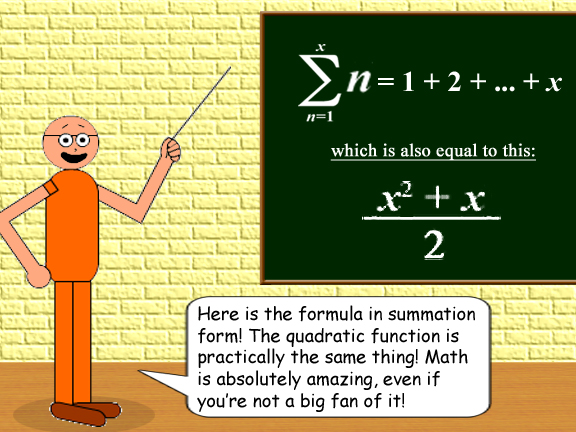# The Formula for the Sum of Consecutive Integers From 1 to x

## To know the sum of consecutive integers, starting from the number 1, you must use & remember this formula:

(x^2 + x)/2 = y

x = the final integer, y = the sum

## Examples:

1 + 2 + 3 + 4 + 5 + 6 + 7 = 28

(7^2 + 7)/2 = (49 + 7)/2 = 56/2 = 28

In this case, x = 7 & y = 28

1 + 2 + 3 + ... + 100 = 5,050

(100^2 + 10)/2 = (10,000 + 100)/2 = 10,100/2 = 5,050

In this case, x = 100 & y = 5,050

If you have a graphing calculator, then you can insert the function at the top of this Web page into your calculator to see the sums in one column(y), and the final integer(s) you chose in the other column(x)! You could also see that it's a quadratic function. Also, notice that the differences of the sums are consecutive integers!1 + 2 + 3 + ... + 250 = 31,375

(250^2 + 250)/2 = (62,500 + 250)/2 = 62,750/2 = 31,375

In this case, x = 250 & y = 31,375## Here's the final & perhaps most interesting example!

1 + 2 + 3 + ... + 666 = 222,111

(666^2 + 666)/2 = (443,556 + 666)/2 = 444,222/2 = 222,111

In this case, x = 666 & y = 222,111To add more info to Goldilocks' comment, if x = 0, then y = 0, according to the function. (Zero is an integer, too!) Furthermore, zero & one are the only 2 idempotent numbers; they're the only 2 numbers that are their own squares.